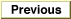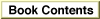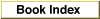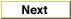Legacy DocumentImportant: The information in this document is obsolete and should not be used for new development.

Inside Macintosh: QuickDraw GX Environment and Utilities /
Chapter 8 - QuickDraw GX Mathematics / QuickDraw GX Mathematics Reference
Mathematical Functions / Fixed-Point Operations

FixedDivide

You can use the FixedDivide function to return the quotient of a dividend and divisor.

Fixed FixedDivide (Fixed dividend, Fixed divisor);
dividend
The number to be divided.
divisor
The number by which the dividend is to be divided.
function result
The quotient of the dividend and the divisor.
DESCRIPTION
The FixedDivide function divides the dividend parameter by the divisor parameter and returns the quotient. The format of the fixed number returned depends on the respective number formats of the dividend and divisor parameters. The operation has a bias of 16 bits; in general, the bias of the resulting number is the difference between the biases of the input numbers, shifted left by 16 bits. If the divisor parameter is fixed, then the result will be the same fixed-point format as the dividend. If both the dividend and divisor are the same fixed-point format, the result will be in Fixed format.

Table 8-4 shows the bias for the quotient of two numbers that are of dissimilar formats. The dashed line indicates that the resulting bias is not equivalent to long, fixed, or fract. Use the rules of the operation to determine it.
FixedDivide quotient bias
DenominatorNumerator
longfixedfract
longfixed------
fixedlongfixedfract
fract------fixed

SPECIAL CONSIDERATIONS
In the case of overflow, FixedDivide pins its result to either the gxPositiveInfinity or gxNegativeInfinity constant.